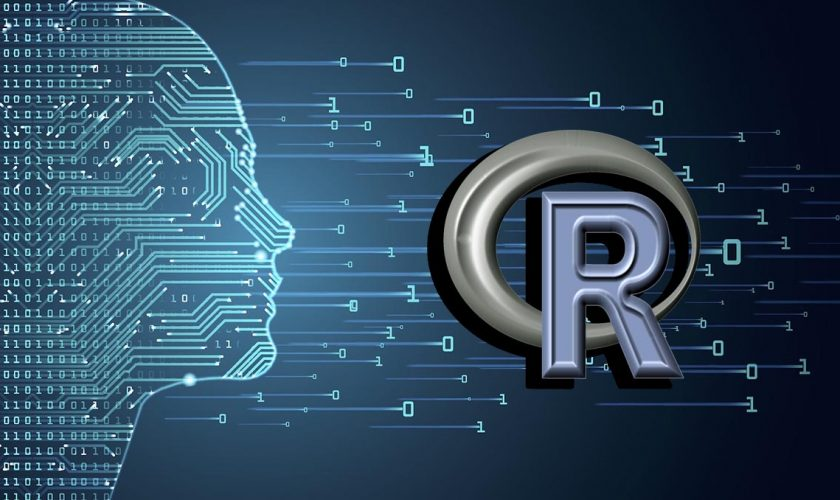• Classroom Training   • Live Online Training   • Corporate Training  • Live Projects & GuidanceISO 9001:2015 Certified

### R Programming###### Courses Information

R is a programming language and free software environment for statistical computing and graphics supported by the R Foundation for Statistical Computing. The R language is widely used among statisticians and data miners for developing statistical software and data analysis.

R is a programming language developed by Ross Ihaka and Robert Gentleman in 1993. R possesses an extensive catalog of statistical and graphical methods. It includes machine learning algorithm, linear regression, time series, statistical inference to name a few. Most of the R libraries are written in R, but for heavy computational task, C, C++ and Fortran codes are preferred.

In this course, you will master the basics of this widely used open source language, including factors, lists, and data frames. With the knowledge gained in this course, you will be ready to undertake your first very own data analysis.

In this course you will learn how to program in R and how to use R for effective data analysis. You will learn how to install and configure software necessary for a statistical programming environment and describe generic programming language concepts as they are implemented in a high-level statistical language.

The course covers practical issues in statistical computing which includes programming in R, reading data into R, accessing R packages, writing R functions, debugging, profiling R code, and organizing and commenting R code. Topics in statistical data analysis will provide working examples.

###### Module 1 - Fundamentals of R Language
• Introduction to Algorithm And Flowcharts
###### Module 2 – Basics of R Language
• General Properties
• First Program - Hello World
###### Module 3 – Data Types in R Language
• Data types introduction
• Data types Examples
###### Module 4 – Variable in R Language
• Introduction to Variable
• Examples on variables
###### Module 5 – Operators in R Language
• Operator Introduction
• Arithmetic Operators
• Relational Operators
• Logical Operators
• Assignment Operators
• Miscellaneous Operators
###### Module 6 – Decision Making of R Language
• Decision Making Introduction
• if statement
• if...else statement
• switch statement
• ifelse() function
###### Module 7 – Loop control in R Language
• Loop Introduction
• for loop
• while Loop
• repeat Loop
• Break Statement
• Next Statement
###### Module 8 – Array in R Language
• Array Introduction
• Array examples
###### Module 9 – String in R Language
• String Introduction
• String examples
###### Module 10 – Functions in R Language
• Functions Introduction
• built-in Function
###### Module 11 – Vector in R Language
• Vector Introduction
• Vector examples
###### Module 12 – Lists in R Language
• List Introduction
• List examples
###### Module 13 – Matrices in R Language
• Matrices Introduction
• Matrix Construction
• Multiplication & Division
• Matrices examples
###### Module 14 – Factors in R Language
• Factors Introduction
• Factors examples
###### Module 15 – Data Frames in R Language
• Data Frame Introduction
• Data Frame details
###### Module 16 – Packages in R Language
• Packages Introduction
• Packages examples
###### Module 17 – Data Reshaping in R language
• Data Reshaping Introduction
• Data Reshaping examples
###### Module 18 – Data and File Management
• Data and File Management
• Types of Input
• CSV Files
• Excel file